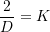### Hyperelastic Materials: A Method for Estimation of the Material Parameters of Hyperelastic Material Models in Relation to the Linear Elastic Material Model

Given a particular form of the strain energy function, we present here a quick method by which the material parameters can be related to the shear modulus and the bulk modulus of a linear elastic material.

### Shear Modulus

The material parameters of a hyperelastic material model can be related to the shear modulus of an elastic material as follows. First, a simple shear state of deformation is assumed: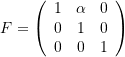The engineering shear strain in this case is equal toand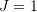. The matrix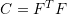is equal to: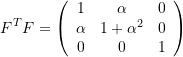The first and second invariants of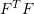are: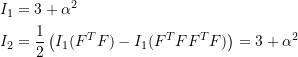The following are examples of some of the compressible and incompressible material models listed above: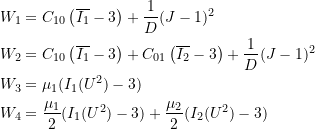For each of these material models, the corresponding Cauchy stress matrix has the form: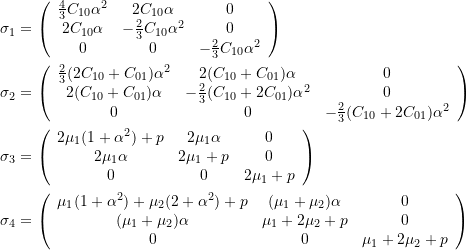For linear elastic materials, the shear stress component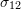and the engineering shear strain component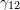are related by the relationship: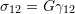. By investigating the componentin the above matrices and by setting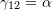, the relationship between the shear modulus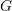and the given material parameters are as follows:

• For material 1: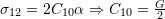• For material 2: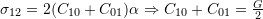• For material 3: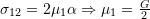• For material 4: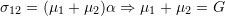### Bulk Modulus

The material parameters of a hyperelastic material model can be related to the bulk modulus of an elastic material as follows. First, a spherical state of deformation is assumed: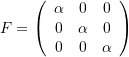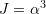. The matrixis equal to: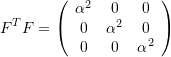The first and second invariants ofare:The following are examples of some of the compressible material models listed above: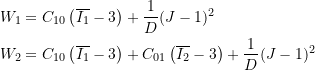For each of these material models, the Cauchy stress has the form: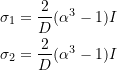For linear elastic materials, the hydrostatic stress component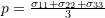is related to the engineering volumetric strain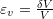by the relationship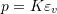. In the deformation described in this problem, the volumetric strain is equal to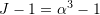, therefore, an estimate of the material constant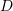for the above materials is given as: